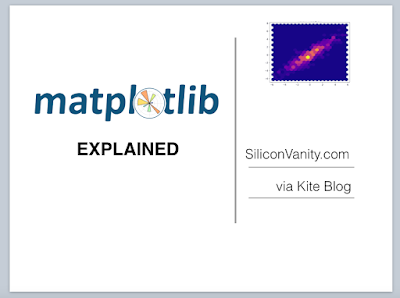## Tuesday, June 26, 2018

### Recurrent Neural Network RNN Basics

Recurrent Neural Network (RNN) is useful for processing sequence data like sound, words, and time series data . RNN remembers a bit of the state from before. It can predict what will come next. Time series is good for heart disease over time, hormone level, blood sugar.

RNN weakness: sometimes gradients too close to 0 or too computationally large. It can also be bad at tracking long term memories - need to use LSTM instead, which has a forget gate, input gate, update layer, output layer

Real world usage:
Transform sequences like text, music, time series data,
Build a RNN generate new text character by character
Natural language processing, Word embedding, Word2Vec model, Semantic relationship between words,
Combine embedding and RNN to predict sentiment of movie reviews

### Hyperparameters in RNN

Hyperparameters are values that we need to set prior to applying an algorithm. Example: learning rate, mini batch size, epochs. There's no magical number. The optimal value depends on the task on hand.

Hyperparameter concepts: starting values, intuitions

Two main types of hyperparameters optimizer hyperparameters and model hyperparameters. Optimizer hyperparameters related to the optimization and training process more than the model itself. Learning rate, mini batch size, number of training iterations are optimizer hyperparameters. Model hyperparameters are variables that relate to the structure of a model. Examples include number of hidden units, number of layers, and model specific hyperparameters.

Yoshua Bengio: learning rate is the most important hyperparameter. Good starting point = 0.01. Also frequently seen: 0.1, 0.01, 0.001, 0.00001, 0.000001

Intuition for starting small (important): If our learning rate is perfect - the multiplier is the best, then in rare ideal scenario we will land at the optimal point. Any learning rate smaller than the perfect rate, will still converge, and would not overshot the optimal point. If learning rate is too large, will never converge (if it is more than twice the optimal rate for example. If it is close enough to the ideal rate, it may still converge. ). Intuition that is must start small. Udacity Deep Learning Nanodegree Part 5 RNN Hyperparameter No.3 Learning Rate gives a great visual illustration.

If learning rate is too small, may take too long to converge, wasting valuable computing resources.